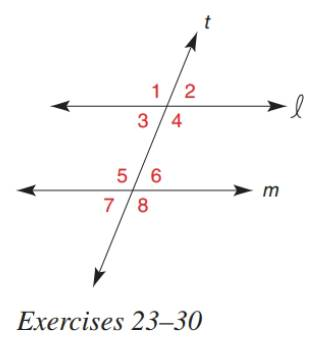Chapter 2.3, Problem 28E### Elementary Geometry for College St...

6th Edition
Daniel C. Alexander + 1 other
ISBN: 9781285195698

#### Solutions

Chapter
Section### Elementary Geometry for College St...

6th Edition
Daniel C. Alexander + 1 other
ISBN: 9781285195698
Textbook Problem
1 views

# In Exercise 23 to 30, determine the value of x so that line l will be parallel to line m . m ∠ 4 = 2 x 2 − 3 x + 6 m ∠ 5 = 2 x ( x − 1 ) − 2To determine

To find:

The value of x for the given statement.

Explanation

Given:

The given statement is,

Line 𝓁 and m are cut by transversal t and are parallel to each other.

m4=2x23x+6 and m5=2x(x1)2.

Figure (1)

Theorem:

If two parallel lines are cut by transversal, then the pairs of alternate interior angles are congruent

Calculation:

Line 𝓁 is parallel to line m.

4 and 5 are alternate interior angles.

So,

### Still sussing out bartleby?

Check out a sample textbook solution.

See a sample solution

#### The Solution to Your Study Problems

Bartleby provides explanations to thousands of textbook problems written by our experts, many with advanced degrees!

Get Started

#### What are the two requirements for a random sample?

Essentials of Statistics for The Behavioral Sciences (MindTap Course List)

#### Evaluate the integral, if it exists. 01(1x9)dx

Single Variable Calculus: Early Transcendentals, Volume I

#### ∫ sin 3x cos 6x dx =

Study Guide for Stewart's Single Variable Calculus: Early Transcendentals, 8th